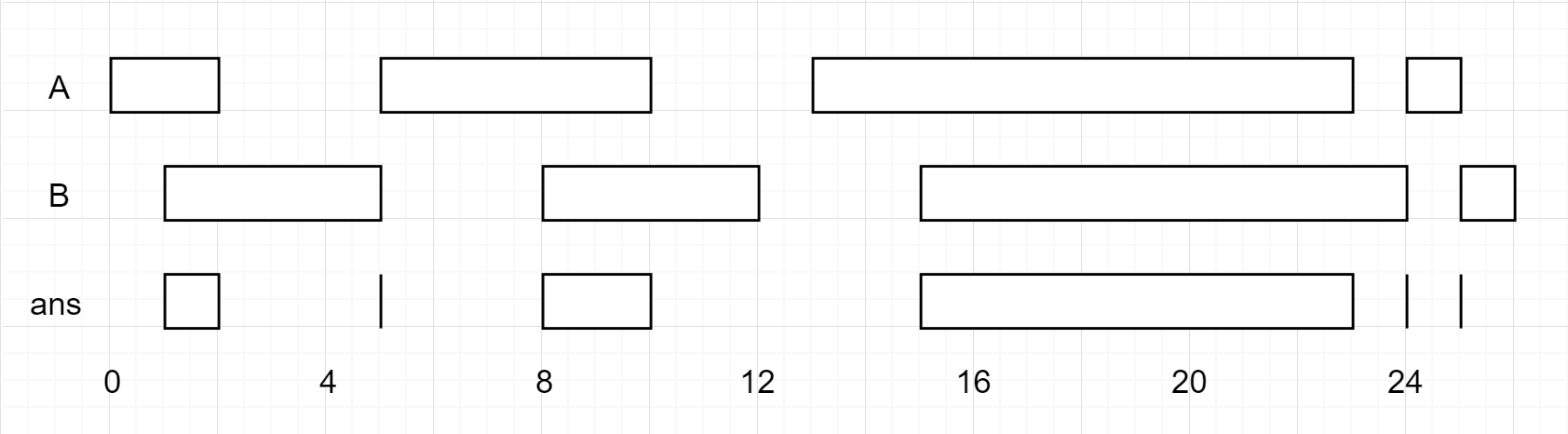# 区间算法问题

Go to the source link to view the article. You can view the picture content, updated content and better typesetting reading experience. If the link is broken, please click the button below to view the snapshot at that time.

## 986. 固定区间的交集1. 判断两个区间是否相交，如果不相交，右侧区间靠前的那一组，索引向后移动
2. 如果相交，取出交集，右侧区间靠前的那一组，索引向后移动
``class Solution {public:    vector<vector<int>> intervalIntersection(vector<vector<int>>& firstList, vector<vector<int>>& secondList) {        int i = 0, j = 0;        vector<vector<int>> res;        while (i < firstList.size() && j < secondList.size()) {            int l1 = firstList[i];            int r1 = firstList[i];            int l2 = secondList[j];            int r2 = secondList[j];            // 没有交集            if (l1 > r2 || r1 < l2) {                // r2 比较小，为了尽快和 l1 相交，需要向后移动                if (l1 > r2) {                    j++;                } else {                    i++;                }                continue;            }            int a = max(l1, l2);            int b = min(r1, r2);            // 交集            res.push_back({a, b});            if (r2 > r1) {                i++;            } else {                j++;            }        }        return res;    }};``

## 1288. 删除覆盖区间

``输入：intervals = [[1,4],[3,6],[2,8]]输出：2解释：区间 [3,6] 被区间 [2,8] 覆盖，所以它被删除了。``

• 如果是覆盖的相交情况，统计数量
• 如果相交的情况，表示上面区间没有能力覆盖下面的区间了，不再考虑上面的区间，开始往下移动
• 如果没有重叠也就是不相交，同样表示上面区间没有能力覆盖下面的区间了，不再考虑上面的区间

``class Solution {public:    static bool cmp(vector<int>& a, vector<int>& b) {        if (a == b)            return a > b;        return a < b;    }int removeCoveredIntervals(vector<vector<int>>& intervals) {        sort(intervals.begin(), intervals.end(), cmp);        int r = intervals;        int cnt = 0;        for (int i = 1; i < intervals.size(); i++) {            // 相交时的处理            if (r >= intervals[i]) {                cnt ++;            }            // 没有覆盖，向后移动            else {                r = intervals[i];            }        }        return intervals.size() - cnt;    }};``

``class Solution {public:    static bool cmp(vector<int>& a, vector<int>& b) {        if (a == b)            return a < b;        return a > b;    }int removeCoveredIntervals(vector<vector<int>>& intervals) {        sort(intervals.begin(), intervals.end(), cmp);        int l = intervals;        int cnt = 0;        for (int i = 1; i < intervals.size(); i++) {            if (l <= intervals[i]) {                cnt ++;            }            else {                l = intervals[i];            }        }        return intervals.size() - cnt;    }};``

## 56. 合并区间

``输入：intervals = [[1,3],[2,6],[8,10],[15,18]]输出：[[1,6],[8,10],[15,18]]解释：区间 [1,3] 和 [2,6] 重叠, 将它们合并为 [1,6].``

• 如果上一个区间覆盖了下一个区间，那么合并区间，也就是相交区间的处理
• 如果上一个区间没有覆盖下一个区间，那么从头开始
``class Solution {public:static bool cmp(vector<int>& a, vector<int>& b) {        if (a == b)            return a > b;        return a < b;    }vector<vector<int>> merge(vector<vector<int>>& intervals) {        sort(intervals.begin(), intervals.end(), cmp);        int r = intervals;        int l = intervals;        vector<vector<int>> res;        for (int i = 1; i < intervals.size(); i++) {            // 相交处理，合并            if (r >= intervals[i]) {                l = min(l, intervals[i]);                r = max(r, intervals[i]);            } else {                // 不能覆盖，先把之前的结果添加进来                // 并重新更新区间                res.push_back({l, r});                l = intervals[i];                r = intervals[i];            }        }        // 最后一组没有被添加进来        res.push_back({l, r});        return res;    }};``

## 452. 用最少数量的箭引爆气球

``输入：points = [[10,16],[2,8],[1,6],[7,12]]输出：2解释：气球可以用2支箭来爆破:-在x = 6处射出箭，击破气球[2,8]和[1,6]。-在x = 11处发射箭，击破气球[10,16]和[7,12]。``

``class Solution {public:static bool cmp(vector<int>& a, vector<int>& b) {        if (a == b)            return a > b;        return a < b;    }int findMinArrowShots(vector<vector<int>>& points) {        sort(points.begin(), points.end(), cmp);        int l = points;        int r = points;        int cnt = 0;        for (int i = 1; i < points.size(); i++) {            if (r < points[i]) {                cnt++;                l = points[i];                r = points[i];            } else {                // 相交处理，有相交区间，缩小相交区间                l = max(l, points[i]);                r = min(r, points[i]);            }        }        return cnt + 1;    }};``

## 435. 无重叠区间

``输入: intervals = [[1,2],[2,3],[3,4],[1,3]]输出: 1解释: 移除 [1,3] 后，剩下的区间没有重叠。``

1. 如果上一个区间覆盖了下面的区间，说明上面的区间太大了。由于我们要删除最少数量的区间，因此首先考虑删除覆盖更广的区间
2. 如果上一个区间和下一个区间相交，此时不能像之前的合并、相交一样，直接移动到下一个区间或处抛弃上面的区间。因为区间在排序后，如果上一个区间和第二个区间相交，上一个区间和第三个区间不相交，此时我们要删除第二个区间，而不是上一个和第三个区间。因此，相交时我们应该保留上一个区间。
``class Solution {public:    static bool cmp(vector<int>& a, vector<int>& b) {        if (a == b)            return a > b;        return a < b;    }    int eraseOverlapIntervals(vector<vector<int>>& intervals) {        sort(intervals.begin(), intervals.end(), cmp);        int r = intervals;        int l = intervals;        int cnt = 0;        for (int i = 1; i < intervals.size(); i++) {            // 覆盖，往下移动            if (l <= intervals[i] && r >= intervals[i]) {                cnt ++;                l = intervals[i];                r = intervals[i];            } else if (r > intervals[i]) {                // 相交，不移动，保留上一个区间                cnt++;            } else {                // 不相交，往后移动，看看后面有多少相交的要处理                r = intervals[i];            }        }        return cnt;    }};``

## 1024. 视频拼接

``输入：clips = [[0,2],[4,6],[8,10],[1,9],[1,5],[5,9]], time = 10输出：3解释：选中 [0,2], [8,10], [1,9] 这三个片段。然后，按下面的方案重制比赛片段：将 [1,9] 再剪辑为 [1,2] + [2,8] + [8,9] 。现在手上的片段为 [0,2] + [2,8] + [8,10]，而这些覆盖了整场比赛 [0, 10]。``

``class Solution {public:    static bool cmp(vector<int>& a, vector<int>& b) {        if (a == b)            return a > b;        return a < b;    }    int videoStitching(vector<vector<int>>& clips, int time) {        sort(clips.begin(), clips.end(), cmp);        int l = 0, r = 0, i = 0, cnt = 0;        // 从起点开始        while (i < clips.size() && clips[i] <= l) {            // 碎片部分和起点相交，选择片段最长的            while (i < clips.size() && clips[i] <= l) {                r = max(clips[i], r);                i++;            }            cnt++;            if (r >= time)                return cnt;            // 最长的片段，是下个片段的起点            l = r;        }        return -1;    }};``